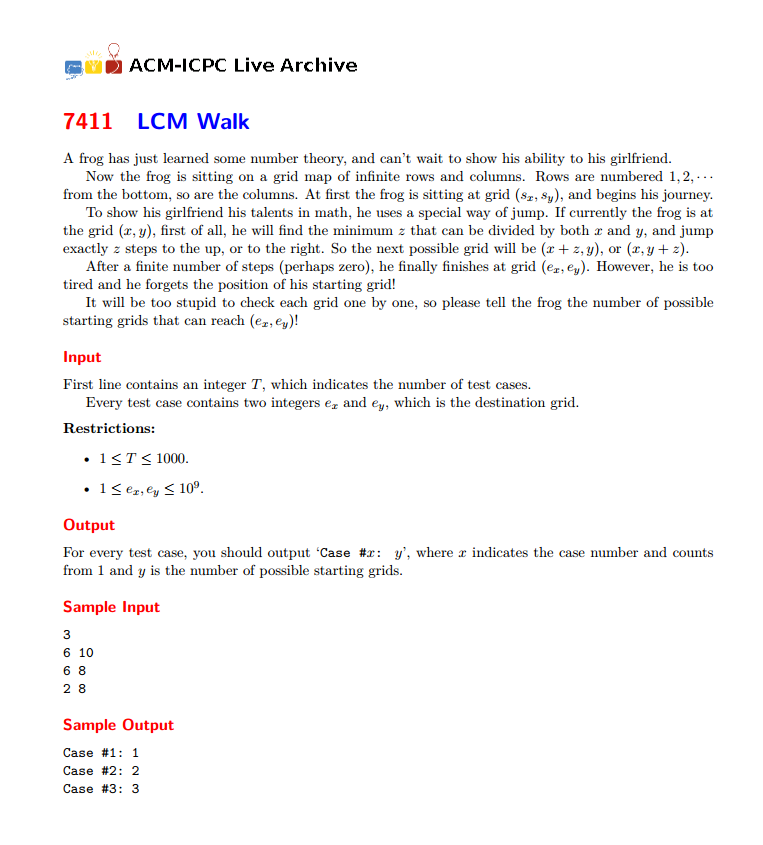# hdu 5584 最小公倍数

## hdu 5584 最小公倍数

2015年ACM区域赛（上海）的现场赛题。

FB（First Blood）时间：18分钟。z是x, y的LCM，设x = pt，y = qt，z = pqt。p和q互质。

（1）终点(ex, ey) = (x, y + z)
= (pt, qt + pqt) = (pt, q(1+p)t)

x = pt = ex
y = qt = ey*t/(ex+t)

（2）终点(ex, ey) = (x + z, y)

#include <bits/stdc++.h>
#define inf 1000000009
#define db printf("where!\n");
#define pb push_back
using namespace std;
#define ll long long
#define MP std::make_pair
ll gcd(ll x,ll y){return y ? gcd(y,x%y) : x;}
char c;T flag=1;
while((c=getchar())<'0'||c>'9')if(c=='-')flag=-1;res=c-'0';
while((c=getchar())>='0'&&c<='9')res=res*10+c-'0';res*=flag;
}

int main()
{
int cnt=0;
while(tt--){
cout<<"Case #"<< ++cnt <<": ";
int ans=1;
if(x>y) swap(x,y);//将小的放前面
while(1){
int t=__gcd(x,y);
int p=x/t;
if(y%(p+1)!=0) break;
int xx=x;
x=y/(p+1),y=xx;
if(__gcd(x,y)!=t) break;
if(x>y) swap(x,y);
ans++;
}
cout<<ans<<endl;
}
return 0;
}

11-29535
11-0644301-17156
11-291528
11-292842
04-13176
04-08725
07-17464
11-21404